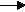gcsescience.com                                       27                                       gcsescience.com

The Periodic Table

The Halogens - Displacement Reactions.

What are Displacement Reactions?

The relative reactivity of the halogens, shown in group trends,
can be shown by displacement reactions.
These are similar to the metal displacement reactions
shown on page 5 of the reactivity series.

For example, when bromine gas is bubbled through a solution
of potassium iodide in water, the more reactive
bromine will displace (take the place of) the less reactive iodide,
forming iodine and potassium bromide.

bromine + potassium iodidepotassium bromide + iodine.
Br2(g)   +        2KI(aq)2KBr(aq)        +     I2(s)

Similarly, chlorine will displace less reactive halogens.
Chlorine is more reactive than both bromine and iodine and
will displace bromine and iodine from the appropriate salt.

Chlorine will displace bromine from sea water.

Chlorine will displace iodine from potassium bromide.

chlorine + potassium iodidepotassium chloride + iodine.
Cl2(g)   +       2KI(aq)2KCl(aq)       +    I2(s)

How can Displacement Reactions be written as Ionic Equations?

When the equations are written in terms of ions
they are called ionic equations. For example, the equation

chlorine + potassium iodidepotassium chloride  +  iodine.
Cl2(g)    +       2KI(aq)2KCl(aq)       +    I2(s)

can be written as

Cl2(g)    +      2I-(aq)2Cl-(aq)     +      I2(s)

Potassium iodide, on the left, exists in solution as
potassium ions (K+) and iodide ions (I-)
and
potassium chloride, on the right, exists in solution as
potassium ions (K+) and chloride ions (Cl-).

Potassium ions (or other metal ions) can be left out of the
ionic equation because they do not change in the reaction.
Ions that are not changed during the reaction can be
called spectator ions, as though they just sit back and watch!

gcsescience.com    The Periodic Table    Index    Periodic Table Quiz    gcsescience.com